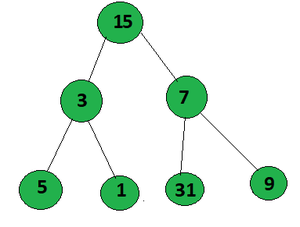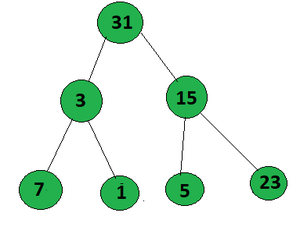# Maximize count of set bits in a root to leaf path in a binary tree

Given a binary tree, the task is to find the total count of set bits in the node values of all the root to leaf paths and print the maximum among them.

Examples:

Input:Output: 12
Explanation:
Path 1: 15(1111)->3(0011)->5(0101) = 8
Path 2: 15(1111)->3(0011)->1(0001) = 7
Path 3: 15(01111)->7(00111)->31(11111) = 12 (maximum)
Path 4: 15(1111)->7(0111)->9(1001) = 9
Therefore, the maximum count of set bits obtained in a path is 12.

Input:Output: 13
Explanation:
Path 1: 31(11111)->3(00011)->7(00111) = 10
Path 2: 31(11111)->3(00011)->1(00001) = 8
Path 3: 31(11111)->15(01111)->5(00101) = 11
Path 4: 31(11111)->15(01111)->23(10111) = 13 (maximum)
Therefore, the maximum count of set bits obtained in a path is 13.

Approach
Follow the steps below to solve the problem:

• Traverse each node recursively, starting from the root node
• Calculate the number of set bits in the value of the current node.
• Update the maximum count of set bits(stored in a variable, say maxm).
• Traverse its left and right subtree.
• After complete traversal of all the nodes of the tree, print the final value of maxm as the answer.

Below is the implementation of the above approach:

## C++

 `// C++ Program to implement ` `// the above approach ` `#include ` `using` `namespace` `std; ` `int` `maxm = 0; `   `// Node structure ` `struct` `Node { ` `    ``int` `val; `   `    ``// Pointers to left ` `    ``// and right child ` `    ``Node *left, *right; `   `    ``// Intialize consutructor ` `    ``Node(``int` `x) ` `    ``{ ` `        ``val = x; ` `        ``left = NULL; ` `        ``right = NULL; ` `    ``} ` `}; `   `// Function to find the maximum ` `// count of setbits in a root to leaf ` `void` `maxm_setbits(Node* root, ``int` `ans) ` `{ ` `    ``// Check if root is not null ` `    ``if` `(!root) ` `        ``return``; `   `    ``if` `(root->left == NULL ` `        ``&& root->right == NULL) { `   `        ``ans += __builtin_popcount(root->val); `   `        ``// Update the maximum count ` `        ``// of setbits ` `        ``maxm = max(ans, maxm); `   `        ``return``; ` `    ``} `   `    ``// Traverse left of binary tree ` `    ``maxm_setbits(root->left, ` `                ``ans + __builtin_popcount( ` `                        ``root->val)); `   `    ``// Traverse right of the binary tree ` `    ``maxm_setbits(root->right, ` `                ``ans + __builtin_popcount( ` `                        ``root->val)); ` `} `   `// Driver Code ` `int` `main() ` `{ ` `    ``Node* root = ``new` `Node(15); ` `    ``root->left = ``new` `Node(3); ` `    ``root->right = ``new` `Node(7); ` `    ``root->left->left = ``new` `Node(5); ` `    ``root->left->right = ``new` `Node(1); ` `    ``root->right->left = ``new` `Node(31); ` `    ``root->right->right = ``new` `Node(9); `   `    ``maxm_setbits(root, 0); `   `    ``cout << maxm << endl; `   `    ``return` `0; ` `} `

## Java

 `// Java Program to implement ` `// the above approach ` `import` `java.util.*;` `class` `GFG{ ` `  `  `static` `int` `maxm = ``0``; `   `// Node structure ` `static` `class` `Node ` `{ ` `    ``int` `val; `   `    ``// Pointers to left ` `    ``// and right child ` `    ``Node left, right; `   `    ``// Intialize consutructor ` `    ``Node(``int` `x) ` `    ``{ ` `        ``val = x; ` `        ``left = ``null``; ` `        ``right = ``null``; ` `    ``} ` `}; `   `// Function to find the maximum ` `// count of setbits in a root to leaf ` `static` `void` `maxm_setbits(Node root, ``int` `ans) ` `{ ` `    ``// Check if root is not null ` `    ``if` `(root == ``null``) ` `        ``return``; `   `    ``if` `(root.left == ``null` `&& ` `        ``root.right == ``null``) ` `    ``{ ` `        ``ans += Integer.bitCount(root.val); `   `        ``// Update the maximum count ` `        ``// of setbits ` `        ``maxm = Math.max(ans, maxm); `   `        ``return``; ` `    ``} `   `    ``// Traverse left of binary tree ` `    ``maxm_setbits(root.left, ` `                ``ans + Integer.bitCount( ` `                        ``root.val)); `   `    ``// Traverse right of the binary tree ` `    ``maxm_setbits(root.right, ` `                ``ans + Integer.bitCount( ` `                        ``root.val)); ` `} `   `// Driver Code ` `public` `static` `void` `main(String[] args) ` `{ ` `    ``Node root = ``new` `Node(``15``); ` `    ``root.left = ``new` `Node(``3``); ` `    ``root.right = ``new` `Node(``7``); ` `    ``root.left.left = ``new` `Node(``5``); ` `    ``root.left.right = ``new` `Node(``1``); ` `    ``root.right.left = ``new` `Node(``31``); ` `    ``root.right.right = ``new` `Node(``9``); `   `    ``maxm_setbits(root, ``0``); `   `    ``System.out.print(maxm +``"\n"``); `   `} ` `} `   `// This code is contributed by Amit Katiyar`

## Python3

 `# Python3 program to implement ` `# the above approach` `maxm ``=` `0`   `# Node class` `class` `Node:` `    `  `    ``# Initialise constructor` `    ``def` `__init__(``self``, x):` `        `  `        ``self``.val ``=` `x` `        ``self``.left ``=` `None` `        ``self``.right ``=` `None` `        `  `# Function to count the number of 1 in number` `def` `count_1(n):` `    `  `    ``count ``=` `0` `    ``while` `(n): ` `        ``count ``+``=` `n & ``1` `        ``n >>``=` `1` `        `  `    ``return` `count `   `# Function to find the maximum ` `# count of setbits in a root to leaf` `def` `maxm_setbits(root, ans):` `    `  `    ``global` `maxm` `    `  `    ``# Check if root is null` `    ``if` `not` `root:` `        ``return` `    `  `    ``if` `(root.left ``=``=` `None` `and` `        ``root.right ``=``=` `None``):` `        ``ans ``+``=` `count_1(root.val)` `        `  `        ``# Update the maximum count ` `        ``# of setbits ` `        ``maxm ``=` `max``(ans, maxm)` `        ``return` `    `  `    ``# Traverse left of binary tree` `    ``maxm_setbits(root.left, ` `                 ``ans ``+` `count_1(root.val))` `    `  `    ``# Traverse right of the binary tree ` `    ``maxm_setbits(root.right, ` `                 ``ans ``+` `count_1(root.val))` `    `  `# Driver code` `root ``=` `Node(``15``)` `root.left ``=` `Node(``3``)` `root.right ``=` `Node(``7``)` `root.left.left ``=` `Node(``5``)` `root.left.right ``=` `Node(``1``)` `root.right.left ``=` `Node(``31``)` `root.right.right ``=` `Node(``9``)`   `maxm_setbits(root, ``0``)`   `print``(maxm)` `        `  `# This code is contributed by Stuti Pathak`

## C#

 `// C# program for the above approach ` `using` `System;` `class` `GFG{` `    `  `// Function to Sort a Bitonic array ` `// in constant space ` `static` `void` `sortArr(``int` `[]a, ``int` `n) ` `{ ` `    ``int` `i, k; `   `    ``// Initialse the value of k ` `    ``k = (``int``)(Math.Log(n) / Math.Log(2)); ` `    ``k = (``int``) Math.Pow(2, k); `   `    ``// In each iteration compare elements ` `    ``// k distance apart and swap if ` `    ``// they are not in order ` `    ``while` `(k > 0) ` `    ``{ ` `        ``for``(i = 0; i + k < n; i++) ` `            ``if` `(a[i] > a[i + k])` `            ``{` `                ``int` `tmp = a[i];` `                ``a[i] = a[i + k];` `                ``a[i + k] = tmp;` `            ``}`   `        ``// k is reduced to half ` `        ``// after every iteration ` `        ``k = k / 2; ` `    ``} `   `    ``// Print the array elements ` `    ``for``(i = 0; i < n; i++)` `    ``{ ` `        ``Console.Write(a[i] + ``" "``); ` `    ``} ` `}` `    `  `// Driver code` `public` `static` `void` `Main(String[] args) ` `{` `    `  `    ``// Given array []arr ` `    ``int` `[]arr = { 5, 20, 30, 40, 36, ` `                  ``33, 25, 15, 10 }; ` `    ``int` `n = arr.Length; ` `    `  `    ``// Function call ` `    ``sortArr(arr, n); ` `}` `}`   `// This code is contributed by gauravrajput1`

Output:

```12

```

Time Complexity: O(N), where N denotes the number of nodes.
Auxiliary Space: O(1)

Attention reader! Don’t stop learning now. Get hold of all the important DSA concepts with the DSA Self Paced Course at a student-friendly price and become industry ready.

My Personal Notes arrow_drop_upCheck out this Author's contributed articles.

If you like GeeksforGeeks and would like to contribute, you can also write an article using contribute.geeksforgeeks.org or mail your article to contribute@geeksforgeeks.org. See your article appearing on the GeeksforGeeks main page and help other Geeks.

Please Improve this article if you find anything incorrect by clicking on the "Improve Article" button below.